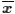## Descriptive Measures

 Note that this page has a dynamic feature to it. The examples on this page will change each time that you load, or reload, the page. Therefore, it may be worth your time to reload the page to see new examples for each of the topics.

We have many ways to describe a collection of data. We will explain and illustrate many such ways in this section.

### Measures of Central Tendency

One of the things that we can do is to try to find a single value, or perhaps a small number of values, that characterize the all of our data points. There are three different "measures" that we use to try to characterize, in the shortest possible way, all of our data. They are the mean, the median and the mode. The following table defines each of these, but here is a link to a much longer web page that goes into much more detail on all three of these measures of central tendency.

### Measures of Dispersion

Besides knowing the "center" of the data, we would also like to have some quick way to get a feel for the "spread" or dispersion of that data. For this discussion we will use the following two example data collections, called A and B:

There is a distinction that should be made at this point. We distinguish between population and sample characteristics by referring to population characteristics as population parameters and sample characteristics as sample statistics. Thus, the mean of a population is a parameters of that population, but the mean of a sample is a statistic of that sample. Beyond that distinction there is no difference in the naming or computing for the measures mode, the median, the range, and the quartiles. The mean of a population, μ, has a different symbol from the one used for the mean of a sample,, however the computation of each is the same. Standard deviation has both different symbols, σ for a population and sx for a sample, and slightly a slightly different formula. That difference will be illustrated in the subsequent web page measures of dispersion.